Welcome to the Onshape forum! Ask questions and join in the discussions about everything Onshape.

First time visiting? Here are some places to start:
1. Looking for a certain topic? Check out the categories filter or use Search (upper right).
2. Need support? Ask a question to our Community Support category.
3. Please submit support tickets for bugs but you can request improvements in the Product Feedback category.
4. Be respectful, on topic and if you see a problem, Flag it.

If you would like to contact our Community Manager personally, feel free to send a private message or an email.

# Understanding inertia

For a part I am interested in the inertia of a crankhaft. I have modeled it, assigned material/density and in the assembly I have added the revolute mate so it turns nicely.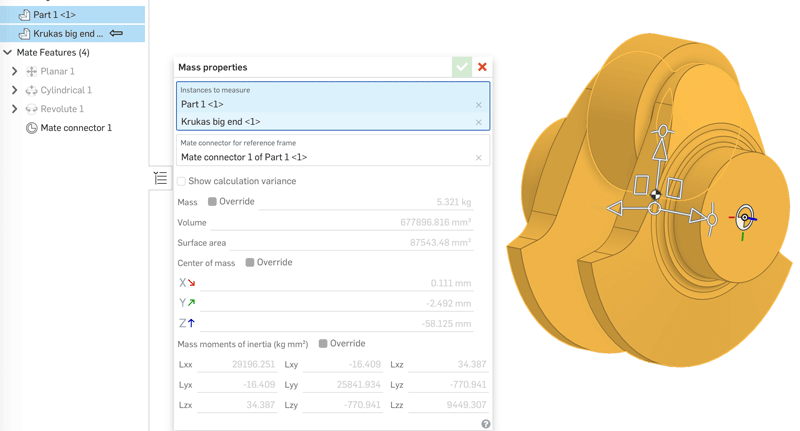I was expecting a single value in kg/m2 for total inertia.
In use the 'Mass Properties Tool' but I do not understand the documentation.
"Moments of inertia - With respect to the common centroid of the selected parts (not the Part Studio origin) and reported using the densities of the materials assigned to the selected parts."
So my question is: How do I add such a common centroid?

And then the next question is, how do I interpret the "Mass moments of inertia" table?
What do these 9 values mean and is there a way to calculate that into the inertia of the model while rotating along the axle?
Thanks,
Regards,
Lars
Tagged:

• My understanding is that inertia properties are initially calculated around the geometric center of your assembly (common centroid). If you have parts with different densities, the centroid may be different than your center of mass. If you want to override this, you can use a mate connector to select a different reference point for your axes. It looks like you've done this already in your screenshot above.

This page has some good info that should help you interpret the table at the bottom (scroll to the second section of the page): https://nrsyed.com/2018/02/06/moments-of-inertia-products-of-inertia-and-the-inertia-tensor/

Essentially, Lxx, Lyy, and Lzz are your moments of inertial around the X,Y, and Z axes, respectively. The off-diagonal values (Lxy, Lxz, etc...) are your "products of inertia". Here's a relevant quote from the page linked above:
Essentially, the moments of inertia tell us how an object rotates about an axis when a torque is applied about that same axis, e.g., if you apply torque about the x axis, Ixx tells us how that affects the angular acceleration about the x axis, based on the mass distribution of the object. The products of inertia tell us how an object rotates about an axis when a torque is applied about a different axis. In the example above, the product of inertia Ixy tells us how a torque about the x axis affects angular acceleration about the y axis, based on the mass distribution of the object. Similarly, Ixz tells us how a torque about the x axis affects angular acceleration about the z axis.

• @lars_rengersen, consider starting with simplified geometry that can easily be verified by hand calculations.  A solid round shaft would be a good starting point.  Then offset the axis of rotation.  That will help to understand the mass properties output.
• @lars_rengersen the mass moments of inertia in the mass properties box don't give you the inertia of the assembly as constrained (i.e. the inertia of a drivetrain accounting for gears, shafts, stationary parts, etc.).

Instead, it gives you the mass moments of inertia around the center of mass with the axes being the assembly's x, y, z axes: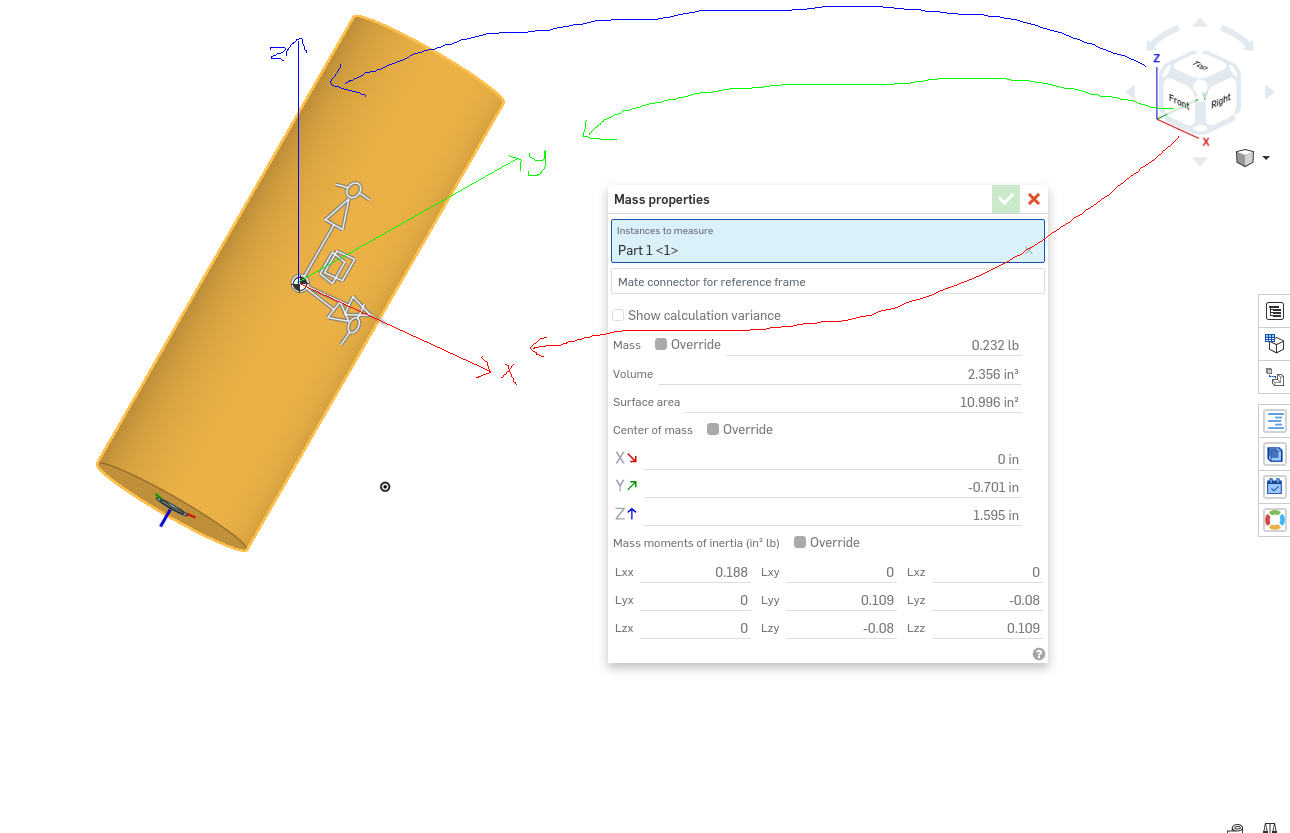You can override the reference frame by specifying the mate connector to use as the (0, 0, 0) point as well as the x, y, z axes: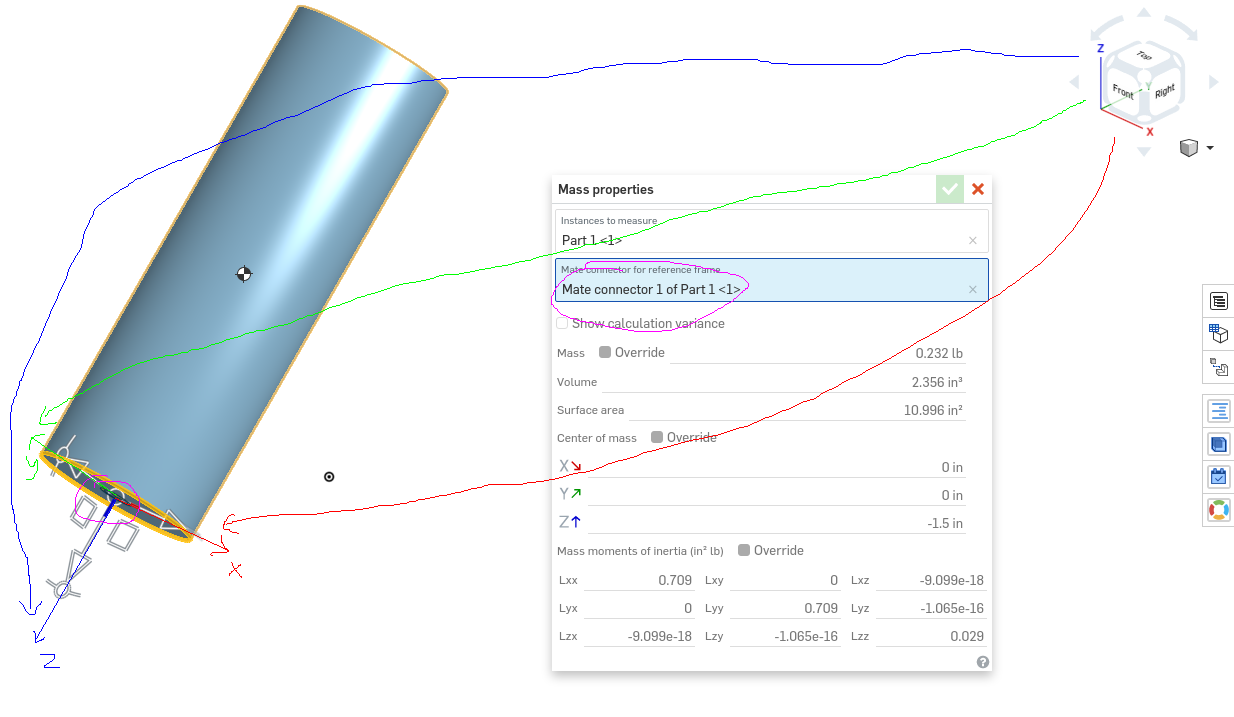I think the documentation should be updated to state that it gives the values with respect to the center of mass rather than the centroid since these are different and it does seem to use the center of mass as the reference point, seeing as manually overriding the reference point to be at the centroid using a mate connector produces different results for the mass moments of inertia calculation (the right cylinder is 10x heavier than the left one):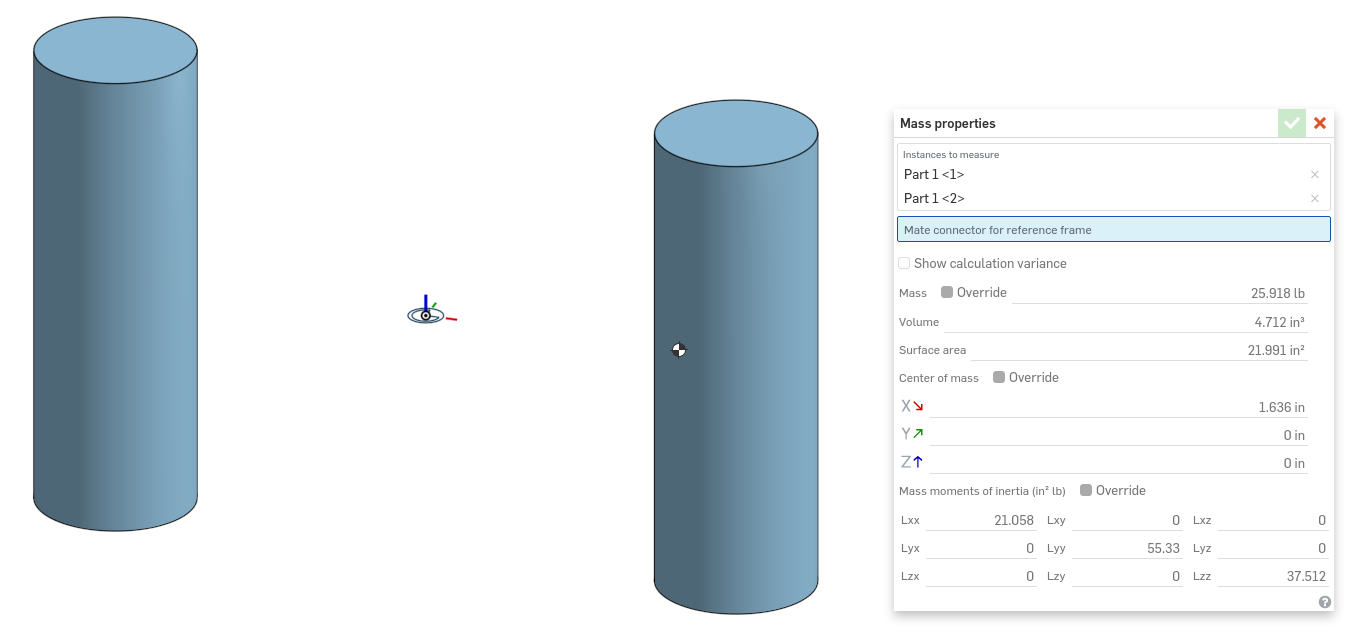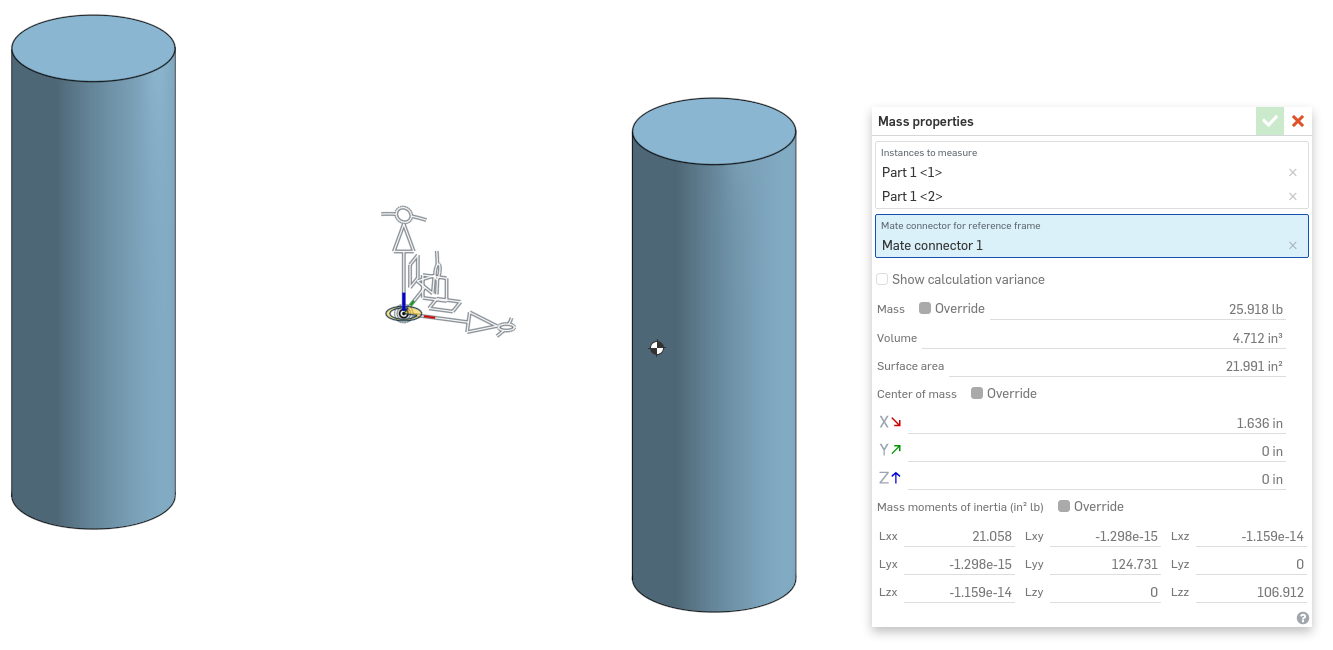Get in touch: [email protected] | My personal site: https://alnis.dev | My YouTube channel (I make tutorial videos for Onshape & Inventor): https://www.youtube.com/c/AlnisSmidchens